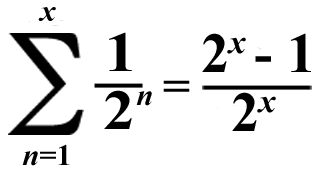# Super Summation 9## The numerator of the sum will always be 1 less than the denominator!

According to the table below, the sum will be equal to these fractions if x is equal to these natural numbers:

 x Sum 1 1/2 2 3/4 3 7/8 4 15/16 5 31/32 6 63/64 7 127/128 8 255/256 9 511/512 10 1023/1024Obviously, the denominator is always equal to a power of 2, but the numerator will be equal to the consecutive integer before that, every time! However, x can't be equal to a number that's less than 1. You can't pick a non-integer for x either, because this is summation.